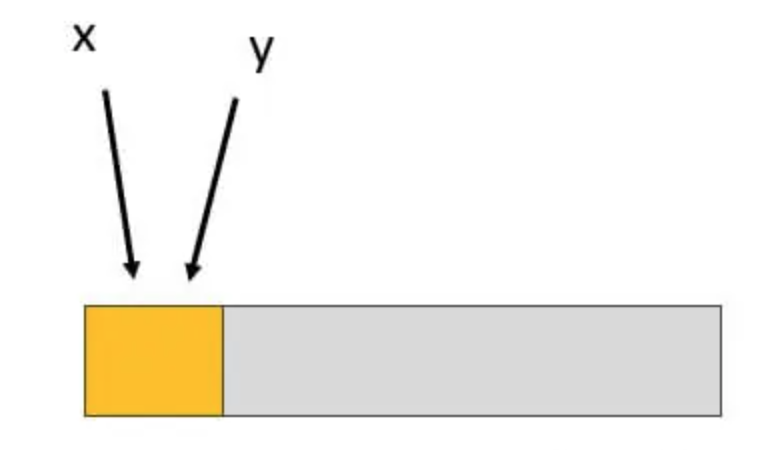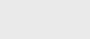2年前 (2022-03-04) |   抢沙发  252

[收起] 文章目录

Java中，当引用（不止一个）链接到同一个对象时，使用Alias（别名）。别名的问题是，当用户写入特定对象时，其他几个引用的所有者不希望该对象发生更改。在这里，包含别名的代码可能会很快变得有点混乱，而且调试起来也非常繁琐。总的来说，别名是一个应该避免或谨慎使用的过程。让我们看看Java Alias是如何工作的。

## alias在Java中是如何工作的？

``````Y[] y = new Y;
X[] x = y;
x =  new X();
Y.func1();````````````Rectangle b1 = new Rectangle (0, 0, 50, 150);
Rectangle b2 = b1;``````

b1和b2都指同一个对象，或者我们可以说给定的对象有两个名称，比如b1和b2。与有两个名字的人类似，对象也可以有两个名字。

``````System.out.println (b2.width);
b1.grow (40, 40);
System.out.println (b2.width);``````

Java别名示例

``````//class X
class X {
//function 1
public void func1()
{
System.out.println("called sample function 1");
}
}
//Class Y that extends the class X
class Y extends X
{
//function 1
public void func1()
{
System.out.println("called sample function 1");
}
//function 2
public void func2()
{
System.out.println("called sample function 2");
}
}
//main class
public class AliasExample {
//main method
public static void main(String[] args) {
Y[] y = new Y;
X[] x = y;
x =  new X();
y.func1();
}
}``````

``````//class X
class X {
//function 1
public void func1()
{
System.out.println("called sample function 1");
}
}
//Class Y that extends the class X
class Y extends X
{
//function 1
public void func1()
{
System.out.println("called sample function 1");
}
//function 2
public void func2()
{
System.out.println("called sample function 2");
}
}
//main class
public class AliasExample {
//main method
public static void main(String[] args) {
Y[] y = new Y;
X[] x = y;
x =  new Y();
y.func1();
}
}``````

``````//main class
public class AliasExample {
//main method
public static void main(String[] args) {
//create two different arrays with same value
int a= 87;
int b=87;
//checks whether a and b are equal
System.out.println(a == b);
//assign b equal to a
b=a;
//checks whether a and b are equal
System.out.println(a == b);
}
}``````

``````//main class
public class AliasExample {
//main method
public static void main(String[] args) {
//create two different arrays with same value
int []a = {81, 54, 83};
int []b = {81, 54, 83};
//checks whether a and b are equal
System.out.println(a == b);
//assign b equal to a
b=a;
//checks whether a and b are equal
System.out.println(a == b);
}
}``````

## 结论### 注册